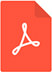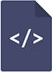# A comparative study between Johnson formula and Hadlock formula for estimating fetal weight in term gestationPDF Full Text

# Abstract

Background: Ultrasound study forms a very important tool in present day obstetrics. The accurate assessment of weight by ultrasound examinations is mandatory for obstetric management particularly at term.

Objective: To compare Johnson’s formula and Hadlock’s formula for estimating fetal weight in term gestation.

Materials and Methods: We performed a prospective observational study in 200 women. The estimated fetal weight (EFW) was measured at term by sonological formula i.e. Hadlock formula [Log10EFW=1.3596-0.00386 (AC x FL)+0.0064 (HC)+0.00061(BPD x AC)+0.0425 (AC)+0.174 (FL)] and Johnson’s formula [(Symphysio-fundal height (cms)-X) * 155] and compared with the actual birth weight. The statistical analysis was done using Z test.

Results: The mean birth weight of Hadlock formula is closest to the mean of actual birth weight when compared to that of Johnson’s formula. P value obtained for both the formulae were <0.01, highly significant. The mean error of the Hadlock formula was 188 grams and the mean error of Johnson formula was 202.148 grams. The percentile error of <20% was 77% in Hadlock formula compared to 79% in Johnson’s formula.

Conclusion: The mean birth weight of hadlock formula was closest to the mean of actual birth weight when compared to Johnson’s formula. The least mean error was noted in the birth weight between 2.5-3.5Kg. Johnson’s formula overestimated the weight in SGA fetuses and Hadlock formula underestimated the weight in LGA fetus.

# Keywords

Estimated fetal weight, Hadlock formula, Johnson’s formula, Ultrasound, Fetal growth retardation, Respiratory distress syndrome, Low birth weight, Abdominal girth, Maternal, Pregnancy, Head circumference, Femur length, Abdominal circumference, Anterior posterior trunk diameter, Transverse trunk diameter